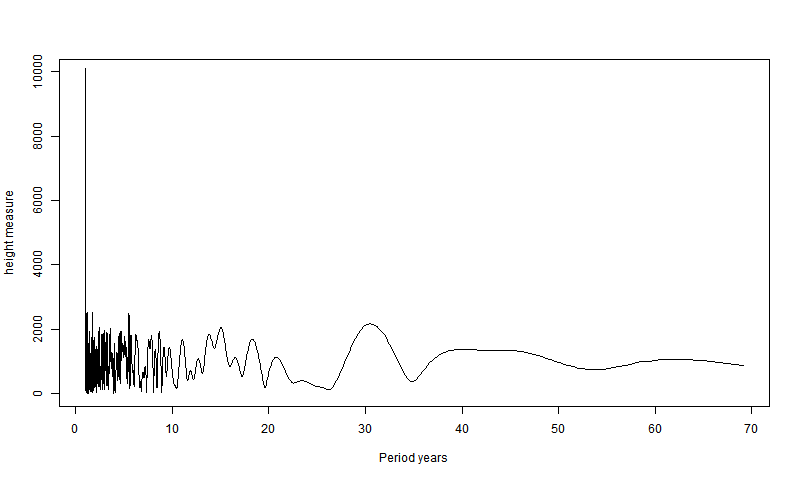Here is my emulation of Willis's first plot of Stockholm data. It needs an extra factor to match the y-axis units. Contrary to my WUWT comment, I have now padded to 2^16 points.

stock.txt is just the CSV file from Willis's XLS with heading text and lines with just ,,, removed. I've put it hereHere is my R program
```v=as.matrix(read.csv("stock.txt"))
a=matrix(NA,227,12)  # First make a mat with 1 row per year
a[v[,1]-1773,]=v[,2:13] # rub out first part with missing years
b=a[-(1:27),]
b=c(t(b))
x=1:length(b)
e=lm(b~x)  # dtrend
b=b-e\$fitted #b=a[-(1:27),]